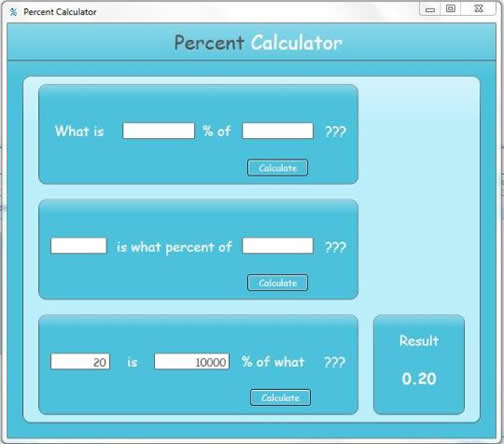# Free Percent Calculator

Percent Calculator is a very user-friendly calculator that makes easy work of percentage calculations. Working out percent equations is easier to do on a calculator than on paper but not much. Even when a calculator is handy, you still have to know how to set up the equation in the first place, before you can enter it into a calculator to solve the equation. But remembering which number it is that is supposed to be divided into which other number is the hard part, because without knowing how to set up the equation, determining the answer to the equation, "20 is what percent of 360" isn't an easy equation to solve, even with a calculator.

Percentage Calculator, on the other hand, is a text based calculator, making the variously phrased percentage calculations a breeze - even for "right-brained" people. Percentage Calculator is a percentage calculator that solves the equations without needing to understand the logistics of mathematical formulas in order to arrive at the correct figures! For instance, trying to determine the answer to "30 percent of 400 = what number?" requires enough mathematical savvy to know that, on a calculator, the equation would be input as "30/100 x 400 =", in order for the calculator to provide the correct answer, which is 30. Percent calculator, on the other hand, phrases the equation just as you would speak it, without the need to translate the question into a mathematical equation first!! This makes percent equations a no-brainer.

Percentage Calculator is an easy, text-based interface that phrases percent questions the way you would encounter them, in everyday life. The user simply provides the needed numbers into the sentence, and then clicks on "calculate" to get the required answer. It really is that easy. And, when percent questions are phrased a different way, these are provided for, also, using the same text-based method. For those of us to whom mathematics never came too easily, Percentage Calculator is the perfect tool for solving percent problems without having to go back and take a course in math.

Percentage Calculator is actually a calculator, like any other calculator, but dedicated to percentage problems and with a much user-friendly interface for the mathematically disinclined. Percentage Calculator addresses the very percentage problems that people encounter, in their everyday life. For instance, let us look at another equation. An example: you are in a store and you are told that you are going to save \$20.00 on an item that retails at 360.00. you may wonder what percent savings you would be getting with the \$20.00 discount. Percentage Calculator addresses this problem with a window that says _ is what percent of _. You just fill in the numbers, click on "calculate" and, Voila! There you have it! 5.56! Percentage Calculator also phrases percent equations one final way: __ is __ % of what number. You simply enter the number 40, for instance, and 20? click on calculate, and there you have it: 200! It really is that easy! Percentage Calculator is the ideal tool for anyone who is bargain shopping, translating the terms of savings (in percents) into actual numbers of dollars and cents!